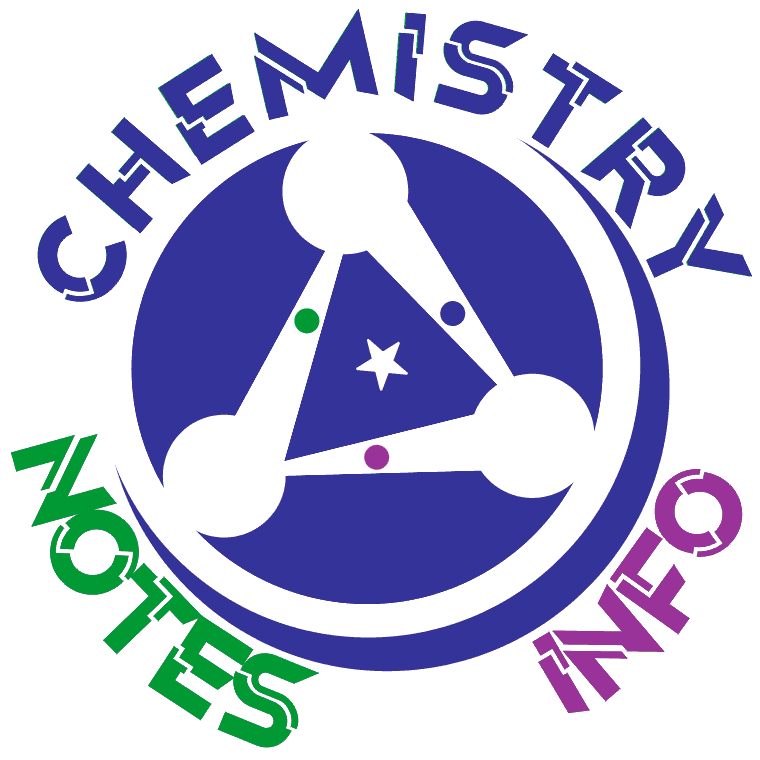NOTE:

If error occur=> Change website url from chemistrynotesinfo.blogspot.in toThe exchange of energy due to difference in temperature is called heat. But as a noun we can say that the Heat is the quality of being hot or high temperature and to understand easily we can say that feeling of hot or warm is heat.

Heat

The exchange of energy due to difference in temperature is called heat. We may also explain as, heat is the form of the energy that is moved between two substances at different temperatures. Means if two substances are in contact and one has 200K temperature and other have 100K temperature then the direction of energy flow is from the substance at the higher temperature to the substance of lower temperature. Means in above case heat flows from substance having 200k temperature to substance having 100K temperature.
Heat is a form of energy so heat is measured in units of energy, commonly calories or joules.

What is Temperature?

Temperature is the quantity of hotness or coldness of any substance. Simply we can say that Temperature is the measurement of how much an object is hot or cold.

Temperature

Temperature is the quantity of hotness or coldness. Also in scientific way, temperature is explained as the average kinetic energy per molecule of a substance. Heat and Temperature (Temp, T) are two different terms and have different meanings so don’t get confuse between them.  Heat is energy while temperature is not energy. Heat is measured in Joules while temperature is measured in Celsius (C), Fahrenheit (F) or Kelvin (K). Symbol used for heat is Q while symbol used for temperature is T. SI unit of heat is Joule while SI unit of temperature is Kelvin.

Heat VS Temperature

Heat is the flow of energy from hot object to cold object while temperature is the measurement of how much an object is hot or cold. Heat is energy while temperature is not energy. Heat is measured in Joules while temperature is measured in Celsius (C), Fahrenheit (F) or Kelvin (K). Symbol used for heat is Q while symbol used for temperature is T. SI unit of heat is Joule while SI unit of temperature is Kelvin.